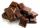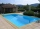# Eggs

3400 eggs sliced hens for February and March. We have to calculate how many hens can make 3400 eggs when one hen give 2 eggs a day for 59 days.

Result

s =  28.814

#### Solution:

3400 = s*2*59

118s = 3400

s = 170059 ≈ 28.813559

Calculated by our simple equation calculator.

Leave us a comment of example and its solution (i.e. if it is still somewhat unclear...):Be the first to comment!#### To solve this example are needed these knowledge from mathematics:

Do you have a linear equation or system of equations and looking for its solution? Or do you have quadratic equation?

## Next similar examples:55%+36%+88%+71%+100=63% what is whole (X)? Percents can be added directly together if they are taken from the same whole, which means they have the same base amount. .. . You would add the two percentages to find the total amount.
2. Two cubesThe surfaces of two cubes, one of which has an edge of 22 cm longer than the second are differ by 19272 cm2. Calculate the edge length of both cubes.
3. Trapezoid MOThe rectangular trapezoid ABCD with right angle at point B, |AC| = 12, |CD| = 8, diagonals are perpendicular to each other. Calculate the perimeter and area of ​​the trapezoid.
4. Right ΔA right triangle has the length of one leg 28 cm and length of the hypotenuse 53 cm. Calculate the height of the triangle.
5. GardenArea of a square garden is 6/4 of triangle garden with sides 56 m, 35 m, and 35 m. How many meters of fencing need to fence a square garden?
6. ChocolateLeslie bought 8 same chocolates for 16 Eur. How many euros will he pay for 25 chocolates?
7. Forestry workersIn the forest is employed 56 laborers planting trees in nurseries. For 8 hour work day would end job in 37 days. After 16 days, 9 laborers go forth? How many days are needed to complete planting trees in nurseries by others, if they will work 10 hours a d
8. BonusGross wage was 527 EUR including 16% bonus. How many EUR were bonuses?
9. Motion problemFrom Levíc to Košíc go car at speed 81 km/h. From Košíc to Levíc go another car at speed 69 km/h. How many minutes before the meeting will be cars 27 km away?
10. Three tributariesIt is possible to fill the pool with three tributaries. The first would take 12 hours, the second 15 hours, and the third 20 hours. The day before the summer season began, the manager opened all three tributaries simultaneously. How long did it take to fil
11. A rectangular patioA rectangular patio measures 20 ft by 30 ft. By adding x feet to the width and x feet to the length, the area is doubled. Find the new dimensions of the patio.
12. A photographA photograph will stick to a white square letter with a x cm length. The photo is 3/4 x cm long and 20 cm wide than the width of the paper. The surface of the remaining paper surrounding the photograph is 990 cm2. Find the size of paper and photo.
13. RT sidesFind the sides of a rectangular triangle if legs a + b = 17cm and the radius of the written circle ρ = 2cm.
14. Six workersSix workers planned to repair the roof in 5 days. After two days, two workers get sick. How long will the remaining workers complete the roof at the same rate of work?
15. Brick weightThe brick weighs 2 kg and a half bricks. How much does one brick weigh?
16. PoolIf water flows into the pool by two inlets, fill the whole for 8 hours. The first inlet filled pool 6 hour longer than second. How long pool take to fill with two inlets separately?
17. TroopsRoute is long 147 km and the first day first regiment went at an average speed 12 km/h and journey back 21 km/h. The second day went second regiment same route at an average speed 22 km/h there and back. Which regiment will take route longer?